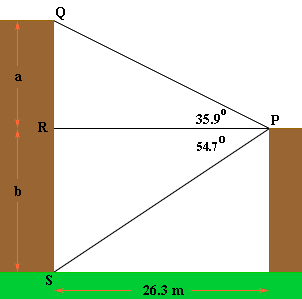Quandaries and Queries I have a problem with trigonometry I was wondering if you could help me. Here it is. Two buildings are 26.3m apart. From the top of the shorter building the angle of elevation to the top of the taller building is 35.9 degrees and the angle of depression to the base of the taller building is 54.7 degrees. What is the height of the taller building? I believe that it is 36.35m tall. What do you think. Nat Hi Nat, I drew a diagram of what I see as the situation.From the diagram the tangent of 35.9 degrees is a/26.3 and the tangent of 54.7 degrees is b/26.3. Hence a = 26.3tan(35.9) = 26.30.7239 = 19.038 b = 26.3tan(54.7) = 26.31.412 = 37.145 Thus the height of the taller building is 19.038 + 37.145 = 56.2 m Penny Go to Math Central